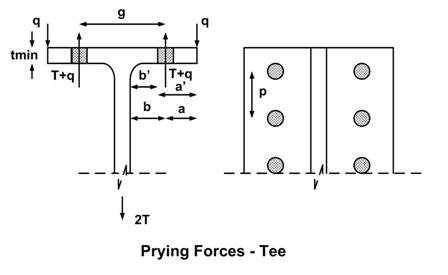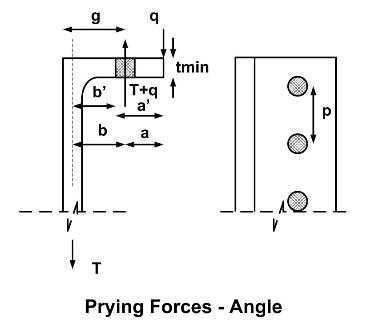* Log In to use the Calculate function * Become a Member!

Calculate Minimum Thickness For Prying Action:

Calculation:
Designer/Checker:

Input:(Note: All input Metric units are converted to English units for the equations below and then the output English units are converted back to Metric)

AISC Chapter 9:
The following is for calculating minimum thickness for prying action of a connection in tension.

Distance from bolt centerline to centerline of angle or to the face of tee stem (b - in or mm)
Required strength per bolt, rut (T - kips or kN)
Bolt diameter (db - in or mm)
Tributary length per pair of bolts for a tee or angle (p - in or mm)  Note:  should not exceed gage, g.
Specified minimum tensile strength of the bolt (Fu - ksi or MPa)

Output: